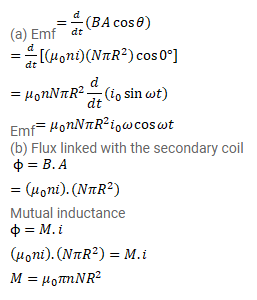# The current in a long solenoid of radius

Question:

The current in a long solenoid of radius $\mathrm{R}$ having $\mathrm{n}$ turns per unit length is given by $i=i_{0} \sin \omega t$. A coil having $\mathrm{N}$ turns is wound around it near the center. Find

(a) the induced emf in the coil and

(b) the mutual inductance between the solenoid and the coil.

Solution: